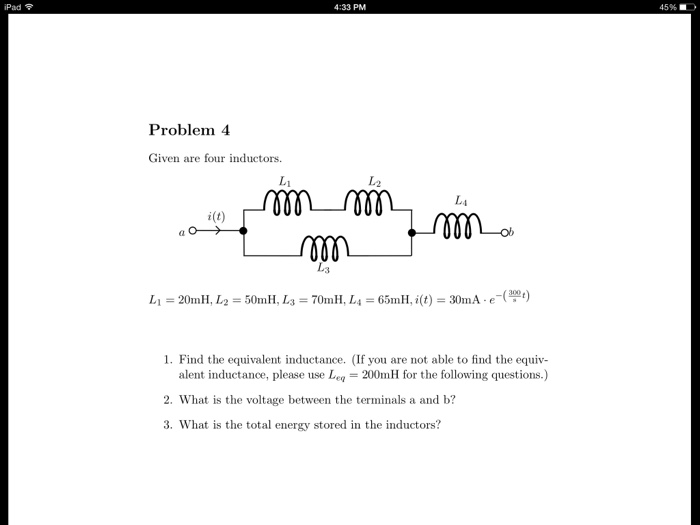# Category: mesh analysis soled poblems – video#### Consider the following circuit. 2 H 2Ω 2HL) a) (1 point) Draw the circuit in the s-domain. b) (2 point) Find the transfer function c) (2 point) Determine the steady state response to the input (2) r(t) = oos(0.10t) + 10000s(1000), t > 0. Problem 2. (3 points) Determine the signal power, rms value and fundamental period of: Icos(0.10t)l 1100cos (1000t) Problem 3. (2 points) The impulse response of a causal discrete-time LTI system is which the zero-state response achieves its maximum. What is this maximum value?

...#### Problem 4 Given are four inductors L1 = 20mH, L2 = 50mH, L3 = 70mH, L4 = 65mH, i(t) = 30mA.e^-(300/s t) 1.Find the equivalent inductance. (if you are not able to find the equivalent inductance please use Leq = 200mH for the following questions.) 2.What is the voltage between the terminals a and b? 3.What is the total energy stored in the inductors?

...#### Find the transfer function, G(s) = F(s)/X2(s), for the translational mechanical system shown in Figure 2.21.

...#### 2.116 Find V x in the network in Fig. P2.116

...#### 2.115 Find Vo in the circuit in Fig. P2.115.

...#### 2.114 Find Io in the circuit in Fig. P2.114

...#### 2.113 Find Io in the circuit in Fig. P2.113.

...#### 2.112 Find in the network in Fig. P2.112

...#### 2.111 Find in the circuit in Fig. P2.111.

...